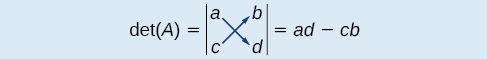# 7.8 Solving systems with cramer's rule

 Page 1 / 11
In this section, you will:
• Evaluate  2 × 2  determinants.
• Use Cramer’s Rule to solve a system of equations in two variables.
• Evaluate  3 × 3  determinants.
• Use Cramer’s Rule to solve a system of three equations in three variables.
• Know the properties of determinants.

We have learned how to solve systems of equations in two variables and three variables, and by multiple methods: substitution, addition, Gaussian elimination, using the inverse of a matrix, and graphing. Some of these methods are easier to apply than others and are more appropriate in certain situations. In this section, we will study two more strategies for solving systems of equations.

## Evaluating the determinant of a 2×2 matrix

A determinant is a real number that can be very useful in mathematics because it has multiple applications, such as calculating area, volume, and other quantities. Here, we will use determinants to reveal whether a matrix is invertible by using the entries of a square matrix to determine whether there is a solution to the system of equations. Perhaps one of the more interesting applications, however, is their use in cryptography. Secure signals or messages are sometimes sent encoded in a matrix. The data can only be decrypted with an invertible matrix and the determinant. For our purposes, we focus on the determinant as an indication of the invertibility of the matrix. Calculating the determinant of a matrix involves following the specific patterns that are outlined in this section.

## Find the determinant of a 2 × 2 matrix

The determinant    of a matrix, given

$A=\left[\begin{array}{cc}a& b\\ c& d\end{array}\right]$

is defined asNotice the change in notation. There are several ways to indicate the determinant, including $\text{\hspace{0.17em}}\mathrm{det}\left(A\right)\text{\hspace{0.17em}}$ and replacing the brackets in a matrix with straight lines, $\text{\hspace{0.17em}}|A|.$

## Finding the determinant of a 2 × 2 matrix

Find the determinant of the given matrix.

$A=\left[\begin{array}{cc}5& 2\\ -6& 3\end{array}\right]$
$\begin{array}{l}\mathrm{det}\left(A\right)=|\begin{array}{cc}5& 2\\ -6& 3\end{array}|\hfill \\ \text{\hspace{0.17em}}\text{\hspace{0.17em}}\text{\hspace{0.17em}}\text{\hspace{0.17em}}\text{\hspace{0.17em}}\text{\hspace{0.17em}}\text{\hspace{0.17em}}\text{\hspace{0.17em}}\text{\hspace{0.17em}}\text{\hspace{0.17em}}\text{\hspace{0.17em}}\text{\hspace{0.17em}}\text{\hspace{0.17em}}\text{\hspace{0.17em}}\text{\hspace{0.17em}}\text{\hspace{0.17em}}=5\left(3\right)-\left(-6\right)\left(2\right)\hfill \\ \text{\hspace{0.17em}}\text{\hspace{0.17em}}\text{\hspace{0.17em}}\text{\hspace{0.17em}}\text{\hspace{0.17em}}\text{\hspace{0.17em}}\text{\hspace{0.17em}}\text{\hspace{0.17em}}\text{\hspace{0.17em}}\text{\hspace{0.17em}}\text{\hspace{0.17em}}\text{\hspace{0.17em}}\text{\hspace{0.17em}}\text{\hspace{0.17em}}\text{\hspace{0.17em}}\text{\hspace{0.17em}}=27\hfill \end{array}$

## Using cramer’s rule to solve a system of two equations in two variables

We will now introduce a final method for solving systems of equations that uses determinants. Known as Cramer’s Rule    , this technique dates back to the middle of the 18th century and is named for its innovator, the Swiss mathematician Gabriel Cramer (1704-1752), who introduced it in 1750 in Introduction à l'Analyse des lignes Courbes algébriques . Cramer’s Rule is a viable and efficient method for finding solutions to systems with an arbitrary number of unknowns, provided that we have the same number of equations as unknowns.

Cramer’s Rule will give us the unique solution to a system of equations, if it exists. However, if the system has no solution or an infinite number of solutions, this will be indicated by a determinant of zero. To find out if the system is inconsistent or dependent, another method, such as elimination, will have to be used.

To understand Cramer’s Rule, let’s look closely at how we solve systems of linear equations using basic row operations. Consider a system of two equations in two variables.

$\begin{array}{c}{a}_{1}x+{b}_{1}y={c}_{1}\text{\hspace{0.17em}}\text{\hspace{0.17em}}\text{\hspace{0.17em}}\text{\hspace{0.17em}}\left(1\right)\\ {a}_{2}x+{b}_{2}y={c}_{2}\text{\hspace{0.17em}}\text{\hspace{0.17em}}\text{\hspace{0.17em}}\text{\hspace{0.17em}}\left(2\right)\end{array}$

how do I set up the problem?
what is a solution set?
Harshika
hello, I am happy to help!
Abdullahi
find the value of 2x=32
divide by 2 on each side of the equal sign to solve for x
corri
X=16
Michael
Want to review on complex number 1.What are complex number 2.How to solve complex number problems.
Beyan
use the y -intercept and slope to sketch the graph of the equation y=6x
how do we prove the quadratic formular
hello, if you have a question about Algebra 2. I may be able to help. I am an Algebra 2 Teacher
thank you help me with how to prove the quadratic equation
Seidu
may God blessed u for that. Please I want u to help me in sets.
Opoku
what is math number
4
Trista
x-2y+3z=-3 2x-y+z=7 -x+3y-z=6
Need help solving this problem (2/7)^-2
x+2y-z=7
Sidiki
what is the coefficient of -4×
-1
Shedrak
the operation * is x * y =x + y/ 1+(x × y) show if the operation is commutative if x × y is not equal to -1
An investment account was opened with an initial deposit of \$9,600 and earns 7.4% interest, compounded continuously. How much will the account be worth after 15 years?
lim x to infinity e^1-e^-1/log(1+x)
given eccentricity and a point find the equiationByBy Brooke DelaneyBy RhodesBy OpenStaxBy OpenStaxBy Karen GowdeyBy OpenStaxBy Rebecca ButterfieldBy Briana HamiltonBy OpenStaxBy OpenStax